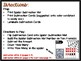# Spider SubtractionSubject
Resource Type
File Type

PDF

(1 MB|44 pages)
Product Rating
4.0
(1 Rating)
Standards
• Product Description
• StandardsNEW

Spider Subtraction is a hands on math center to target Subtraction within 10 and within 20.

Spider Subtraction Prep:

• Print Spider Subtraction Mat
• Print Subtraction Cards (suggested: onto color cardstock to easily see.)
• Laminate Subtraction Cards

Spider Subtraction Prep/Directions:

• Flip Subtraction Card onto Spider Subtraction Mat.
• Build the Spider Subtraction Mat Number on Ten Frame(s).
• Subtract the Amount.
• Count ‘How Many’.
• Write ‘How Many’ in the Spider.

1. Subtraction Cards within 10
2. Spider Subtraction Mat (10 Frame)
3. Subtraction Cards within 20
4. Spider Subtraction Mat (Double 10 Frame)
Add and subtract within 20, demonstrating fluency for addition and subtraction within 10. Use strategies such as counting on; making ten (e.g., 8 + 6 = 8 + 2 + 4 = 10 + 4 = 14); decomposing a number leading to a ten (e.g., 13 - 4 = 13 - 3 - 1 = 10 - 1 = 9); using the relationship between addition and subtraction (e.g., knowing that 8 + 4 = 12, one knows 12 - 8 = 4); and creating equivalent but easier or known sums (e.g., adding 6 + 7 by creating the known equivalent 6 + 6 + 1 = 12 + 1 = 13).
Apply properties of operations as strategies to add and subtract. If 8 + 3 = 11 is known, then 3 + 8 = 11 is also known. (Commutative property of addition.) To add 2 + 6 + 4, the second two numbers can be added to make a ten, so 2 + 6 + 4 = 2 + 10 = 12. (Associative property of addition.)
Solve addition and subtraction word problems, and add and subtract within 10, e.g., by using objects or drawings to represent the problem.
Total Pages
44 pages
N/A
Teaching Duration
N/A
Report this Resource to TpT
Reported resources will be reviewed by our team. Report this resource to let us know if this resource violates TpT’s content guidelines.